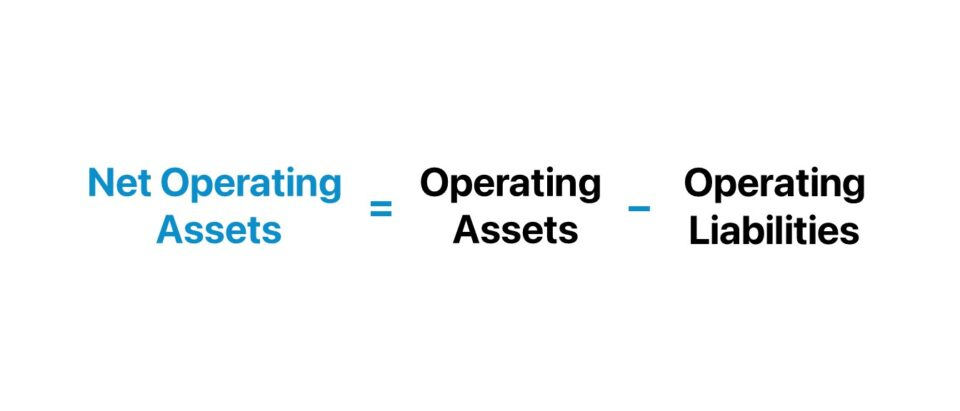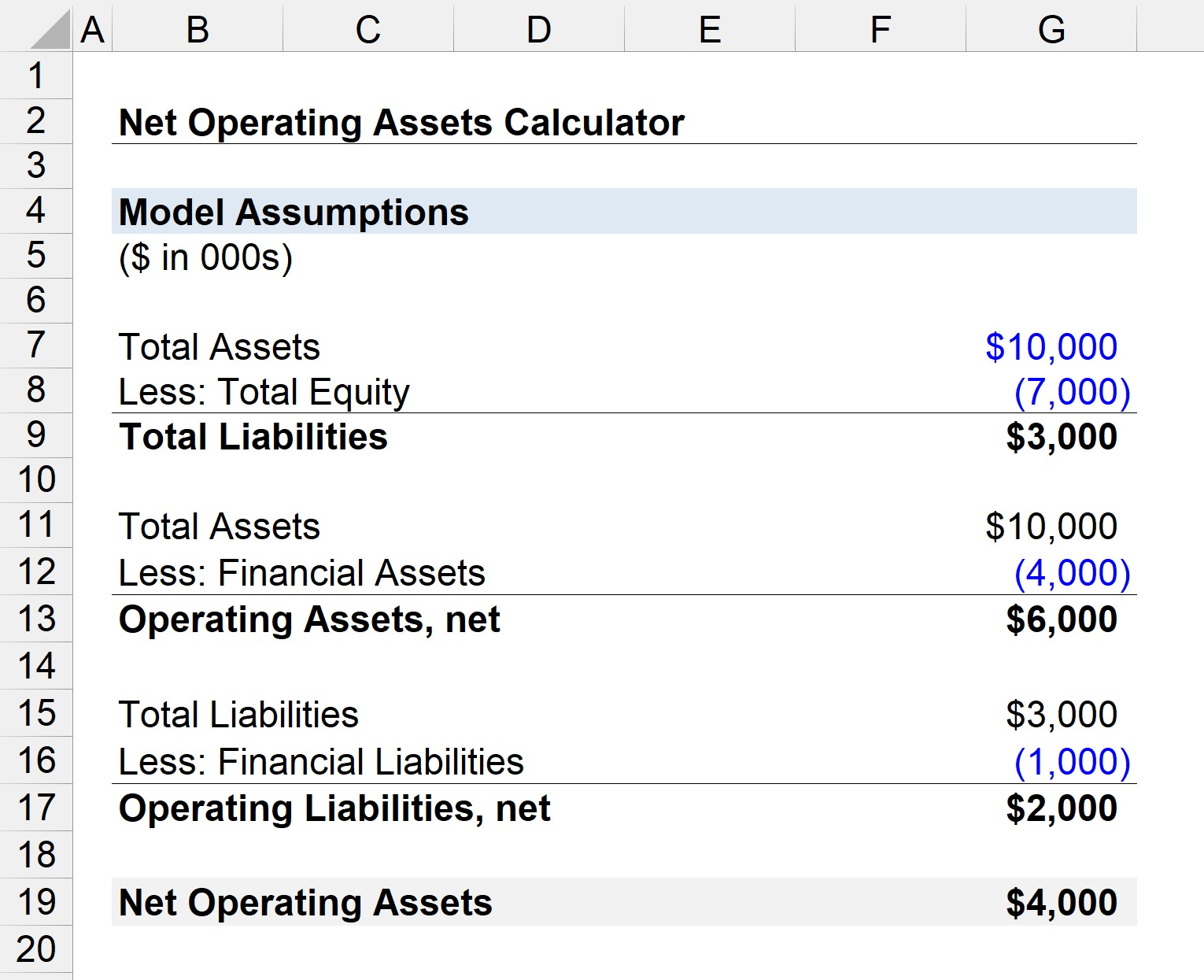Welcome to Wall Street Prep! Use code at checkout for 15% off.# Net Operating Assets

Guide to Understanding Net Operating Assets## How to Calculate Net Operating Assets?

The net operating assets (NOA) of a company equal the value of all assets directly tied to core operations minus all operational liabilities.

• Operating Asset → If an asset is needed for a company’s operations to continue running, it would most likely be considered an “operating asset”.
• Operating Liability → If a certain liability is necessary for a company’s operations to sustain itself, it would be classified as an “operating liability”.

Common examples of operating assets and operating liabilities are as follows.

Operating Assets Operating Liabilities

## Net Operating Assets Formula

The formula to calculate net operating assets (NOA) subtracts operating liabilities from operating assets.

Net Operating Assets = Operating Assets Operating Liabilities

Therefore, the net operating assets belonging to a company represent the difference between its operating assets and operating liabilities.

Operating assets and operating liabilities both support the continuation of revenue production from core operations.

• Operating Assets: The assets of a company required for its core operations to continue functioning (e.g. inventory and the production of products to sell).
• Operating Liabilities: The liabilities of a company that are part of the day-to-day operations (e.g. accounts payable and supplier orders).

## Operating vs. Non-Operating Items: What is the Difference?

An example of an asset that does NOT qualify as an operating asset would be marketable securities, which would be classified as an investing activity.

Despite the short-term investment creating income for the company, it is considered “side income” and a non-core asset unrelated to its core, recurring operations.

Further, an example of a liability that would NOT qualify as an operating liability would be long-term debt, as debt is a financing activity.

The value of a company’s operating assets is equal to the sum of all operating assets less the value of all non-operating assets.

Operating Assets, net = Operating Assets Non-Operating Assets

Similarly, the value of a company’s operating liabilities is equal to the sum of all operating liabilities less the value of all non-operating liabilities.

Operating Liabilities, net = Operating Liabilities Non-Operating Liabilities

## Net Operating Assets Calculator

We’ll now move to a modeling exercise, which you can access by filling out the form below.Submitting...

## Net Operating Assets Calculation Example

Suppose a company has \$10 million in total assets and total shareholders equity of \$7 million on its balance sheet.

• Total Assets = \$10 million
• Total Equity = \$7 million

Of the \$10 million in assets, \$4 million is related to financial assets such as marketable securities and short-term investments.

We can subtract the non-operating assets from total assets to calculate \$6 million as the company’s total operating assets.

• Operating Assets, net = \$10 million – \$4 million = \$6 million

If the company has \$1 million in outstanding long-term debt on its books, we can subtract this amount from its total liability balance.

• Financial Liabilities = \$1 million

Considering the accounting equation (assets = liabilities + equity), the total liabilities must be \$3 million, given the \$10 million in assets and the \$7 million in equity.

Once the \$1 million in debt is subtracted from the \$3 million in total liabilities, we are left with \$2 million as the operating liabilities.

• Operating Liabilities, net = \$3 million – \$1 million = \$2 million

Using those two values, we can subtract the operating liabilities from operating assets to arrive at the value for net operating assets, which is \$4 million.

• Net Operating Assets = \$6 million – \$2 million = \$4 millionStep-by-Step Online Course

### Everything You Need To Master Financial Modeling

Enroll in The Premium Package: Learn Financial Statement Modeling, DCF, M&A, LBO and Comps. The same training program used at top investment banks.

Inline Feedbacks